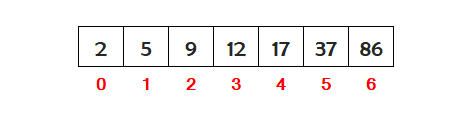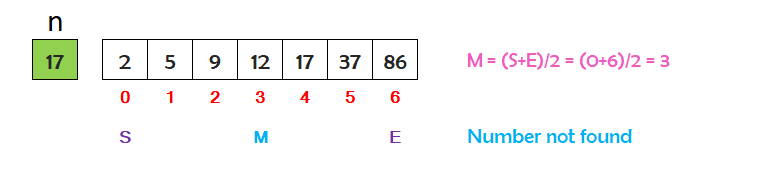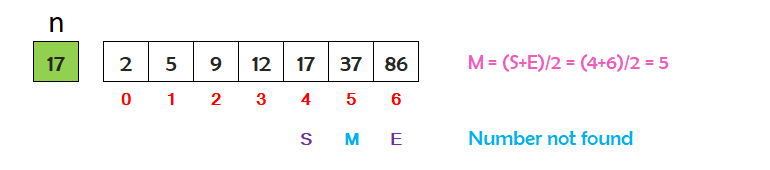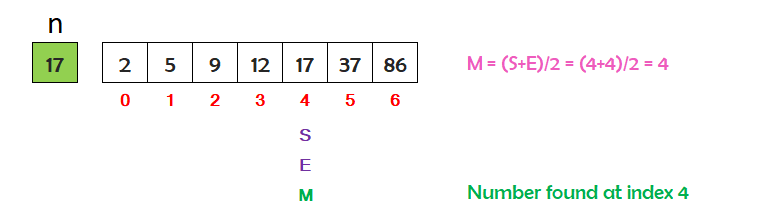# Binary Search in C Programming

###### Searching in C

In this lesson, we will understand what is Binary Search in C Programming along with some examples.

## What is Binary Search in C?

A Binary Search is a searching technique used in C programming to search an element from an array. Binary search only works on sorted arrays.

Suppose we have a sorted array in ascending order, and we are looking for an element in the array, which is situated, at the end of the array. In this case, linear search is not suitable because it will scan each element from the start until it reached the last element that we are searching for in the array. In this situation, Binary Search is a more suitable and efficient searching technique compare to Linear Search.

In this searching technique, we compare the search element with the middle element of the array. If the search element is greater than the middle element, then we start searching in the right portion of the array after the middle element. If the search element is less then the middle element, then we start searching in the left portion of the array below the middle element. Now we will take only that portion in which the search element may be present and again compare the search element with the middle element of that portion. This process will be in iteration until we find the element, or there is no left or right portion left to search the element.

To clearly understand the binary search technique, let's go through its algorithm step by step with an example.## Binary Search Visualization

Suppose we have seven numbers stored in ascending order in an array as shown below. We want to search the number 17 in the array.n is a variable that contains the number 17 we want to search in the array.

S is a variable stands for start index and contains the index 0.

E is a variable stands for end index and contains the index 6.

M is a variable stands for middle index and contains the index (S+E)/2=3.

The value of n=17 is not matching with the middle index value 12. The value of n is greater then the middle index value. So we will search in the right portion of the array starting after the middle index.Now the value of S will be M+1=4.

The value of E remains the same, that is 6.

The value of M will be (S+E)/2=5.

The value of n=17 is not matching with the middle index value 37. The value of n is smaller then the middle index value. So we will search in the left portion of the array below the middle index.The value of S remains same, that is 4.

Now the value of E will be M-1=4.

The value of M will be (S+E)/2=4.

Now the value of n=17 is matching with the middle index value 17. So, the search is successful and the element is found at index 4.

## Algorithm for Binary Search

An algorithm is the steps used to implement the binary search in a c program.

Assume we have N numbers in ascending order, stored in an array. To implement the binary search on N numbers, the steps are as follows.

• Define an array to store N numbers in ascending order for binary search. Suppose we have defined an array with the name num.
• Store the number we want to search in a variable say x.
• Declare a variable f and set its value 0. For example f=0.
• Set the value of the start index variable S=0 and end index variable E=N-1.
• Run a while loop i till S<=E.
• Find the middle index M by dividing the sum of S and E by 2 and consider only the integer part of the result.
• Check if value of x is equal to the value of num[M] then print message Number found at index along with the value of M and set f=1 and then break the loop.
• Check If value of x is greater than the value at num[M] then set the start index S=M+1.
• If value of x is smaller than the value at num[M] then set the end index E=M-1.
• Outside the body of the loop i check if value of f is 0 then print the message Number not found.

## C Program for Binary Search

A C program to search a number in an array having numbers in ascending order using binary search technique.

#### Binary Search when array is in ascending order

``````#include <stdio.h>
#include <conio.h>

int main()
{
// define an array that contain numbers in ascending order
int num[]= {2,5,9,12,17,37,86};
int i,x,f,S,E,M;

printf("Array: ");
for(i=0; i<7; i++)
{
printf("%d ",num[i]);
}

// store the number in variable x to search in the array
printf("\n\nEnter number to search ");
scanf("%d",&x);

// set the value of variable f=0
f=0;

// set the start index S, end index E with the start and end index of the array
S=0;
E=6;

// run a while loop till S is less than or equal to E
while(S<=E)
{
// find the middle index M by dividing the sum of S and E by 2
// and consider only the integer part of the result.
M=(S+E)/2;

// check if value of x is equal to the value of num[M] then print message
// 'Number found at index' along with the value of M and set f=1 and then break the loop
if(x==num[M])
{
printf("Number found at index %d",M);
f=1;
break;
}
else if(x>num[M])  // check if value of x is greater than the value at num[M] then set the start index S=M+1
{
S=M+1;
}
else if(x<num[M])  // check if value of x is smaller than the value at num[M] then set the end index E=M-1
{
E=M-1;
}
}

// check if value of f is 0 then print number not found
if(f==0)
{
}
return 0;
}``````

#### Output

```Array: 2 5 9 12 17 37 86

Enter number to search 17
Number found at index 4```

A C program to search a number in an array having numbers in descending order using binary search technique.

#### Binary Search when array is in descending order

``````#include <stdio.h>
#include <conio.h>

int main()
{
// define an array that contain numbers in ascending order
int num[]= {86,37,17,12,9,5,2};
int i,x,f,S,E,M;

printf("Array: ");
for(i=0; i<7; i++)
{
printf("%d ",num[i]);
}

// store the number in variable x to search in the array
printf("\n\nEnter number to search ");
scanf("%d",&x);

// set the value of variable f=0
f=0;

// set the start index S, end index E with the start and end index of the array
S=0;
E=6;

// run a while loop till S is less than or equal to E
while(S<=E)
{
// find the middle index M by dividing the sum of S and E by 2
// and consider only the integer part of the result.
M=(S+E)/2;

// check if value of x is equal to the value of num[M] then print message
// 'Number found at index' along with the value of M and set f=1 and then break the loop
if(x==num[M])
{
printf("Number found at index %d",M);
f=1;
break;
}
else if(x>num[M])  // check if value of x is greater than the value at num[M] then set the end index E=M-1
{
E=M-1;
}
else if(x<num[M])  // check if value of x is smaller than the value at num[M] then set the start index S=M+1
{
S=M+1;
}
}

// check if value of f is 0 then print number not found
if(f==0)
{
}
return 0;
}``````

#### Output

```Array: 86 37 17 12 9 5 2

Enter number to search 17
Number found at index 2```

## Binary Search Program Explanation

The explanation of the above program is given below in details.

#### Explanation

```Array: 2 5 9 12 17 37 86

x = 17  Number to be search in the array
f = 0

S = 0   Start index
E = 6   End index

Running a while loop i till S<=E

When S = 0 and E = 6
M = (S+E)/2 = 3
Value of x=17 and num=12
Check if 17==12   17 is greater than 12
So Start index will be S=M+1 = 4
Now we will search 17 between index 4 and 6

When S = 4 and E = 6
M = (S+E)/2 = 5
Value of x=17 and num=37
Check if 17==37   17 is smaller than 37
So End index will be E=M-1 = 4
Now we will search 17 between index 4 and 4

When S = 4 and E = 4
M = (S+E)/2 = 4
Value of x=17 and num=17
Check if 17==17  Yes
Number found at index 4

f = 1
Break the loop

Loop i ends

Check if f==0   No```